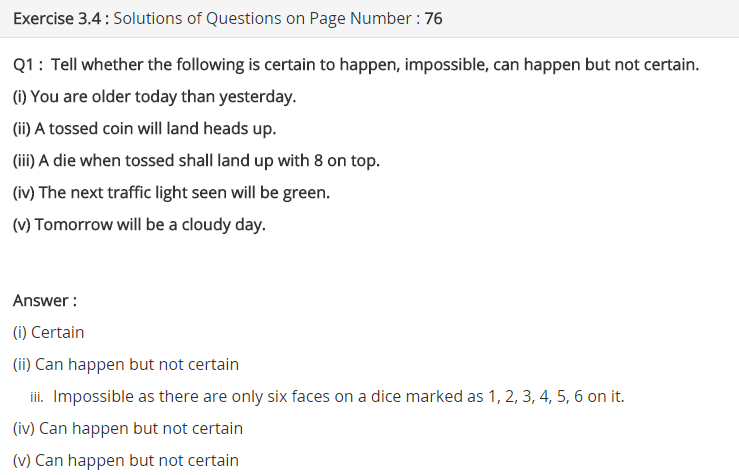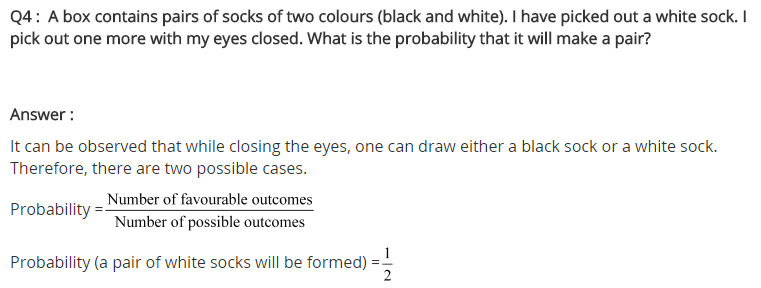# NCERT Solutions for Class 7 Maths Chapter 3 Data Handling Ex 3.4

NCERT Solutions for Class 7 Maths Chapter 3 Data Handling Ex 3.4

### NCERT Solutions for Class 7 Maths Chapter 3 Data Handling Ex 3.4

NCERT Solutions for Class 7 Maths Chapter 3 Data Handling Exercise 3.4
Ex 3.4 Class 7 Maths Question 1.
Tell whether the following situations are certain to happen, impossible to happen, can happen but not certain.
(i) You are older today than yesterday.
(ii) A tossed coin will land heads up.
(iii) A dice when tossed shall land up with 8 on top.
(iv) The next traffic light seen will be green.
(v) Tomorrow will be a cloudy day.
Solution:

 Event Chance (i) You are older today than yesterday. Certain to happen. (ii) A tossed coin will land heads up. Can happen but not certain. (iii) A dice when tossed shall land up 8 on the top. Impossible. (iv) The next traffic light seem will be green. Can happen but not certain. (v) Tomorrow will be cloudy day. Can happen but not certain.

Ex 3.4 Class 7 Maths Question 2.
There are 6 marbles in a box with numbers from 1 to 6 marked on each of them.
(i) What is the probability of drawing a marble with number 2?
(ii) What is the probability of drawing a marble with number 5?
Solution:
(i) Total number of marbles marked with the number from 1 to 6 = 6
∴ n(S) = 6
Number of marble marked with 2=1
∴ n(E) = 1Ex 3.4 Class 7 Maths Question 3.
A coin is flipped to decide which team starts the game. What is the probability that your team will start?
Solution:
Coin has 2 faces—Head (H) and Tail (T)
∴ Sample space S(n) = 2
Number of successful event n(E) = 1Sample Space: The sample space of an experiment is the number of all possible outcomes of that experiment.## SabDekho

The Complete Educational Website